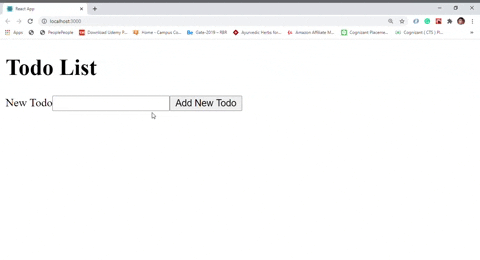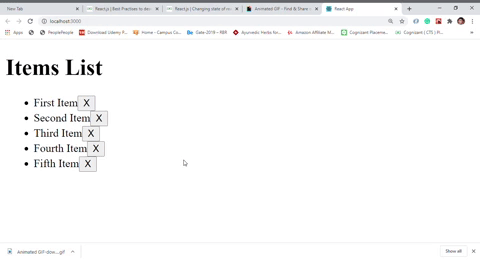# How to handle states of mutable data types?

• Last Updated : 30 Sep, 2020

Mutable parameters are those whose value can be modified within a function to which they are passed as a parameter. It means that when a parameter is passed to the function using the caller function, then its value is bound to the parameter in the called function, which means any changes done to the value in that function will also be reflected in the parameter of the caller function.

The state is mutable in react components. To make the React applications interactive we almost use state in every react component. State is initialized with some value and based on user interaction with the application we update the state of the component at some point of time using the setState method. If states of the React component initialized with an array or JavaScript object, It is always good and recommended practice to not change the state by modifying the old array or object itself but use some build-in JavaScript method like a map, filter, or use new JavaScript syntax like spread to return the newly updated state as a whole.

Example 1: In this example, we will create a todo app, where the user will interact and store the things he or she wants to do, which is basically a mutable state.

• index.js:

## Javascript

 `import React from ``'react'` `import ReactDOM from ``'react-dom'` `import App from ``'./App'` ` `  `ReactDOM.render(, document.querySelector(``'#root'``))`

• App.js :

## Javascript

 `import React from ``'react'` `import TodoList from ``'./TodoList'` ` `  `const App = () => { ` `  ``return` ` ` `} ` ` `  `export ``default` `App`

• TodoList.js :

## Javascript

 `import React, { Component } from ``'react'` `import TodoForm from ``'./TodoForm'` ` `  `class TodoList extends Component{ ` `  ``constructor(props){ ` `    ``super``(props) ` `    ``this``.state = { todos : [] } ` `    ``this``.createTodo = ``this``.createTodo.bind(``this``) ` `    ``this``.renderTodos = ``this``.renderTodos.bind(``this``) ` `  ``} ` ` `  `  ``createTodo(todo){ ` `    ``this``.setState({ ` `     `  `      ``// Changing state with returning new list of todos ` `      ``// as a whole not modyfying the old list of todos ` `      ``todos : [...``this``.state.todos, todo] ` `    ``}) ` `  ``} ` ` `  ` `  `  ``renderTodos(){ ` `    ``const todos = ``this``.state.todos.map(todo => ( ` `      ``
• ` `        ``{todo.task} ` `      ``
• ` `    ``)) ` `    ``return` `
{todos}
` `  ``} ` ` `  `  ``render(){ ` `    ``return``( ` `      ``
` `        ``

Todo List

` `        ``{``this``.renderTodos()} ` `        `` ` `      ``
` `    ``) ` `  ``} ` `} ` ` `  `export ``default` `TodoList`

• TodoForm.js :

## Javascript

 `import React, { Component } from ``'react'` ` `  `class TodoForm extends Component{ ` `  ``constructor(props){ ` `    ``super``(props) ` ` `  `    ``this``.state = { task:``''` `} ` `    ``this``.handleChange = ``this``.handleChange.bind(``this``) ` `    ``this``.handleSubmit = ``this``.handleSubmit.bind(``this``) ` `  ``} ` ` `  `  ``handleChange(event){ ` `    ``this``.setState({ ` `      ``[event.target.name] : event.target.value ` `    ``}) ` `  ``} ` ` `  `  ``handleSubmit(event){ ` `    ``event.preventDefault() ` `    ``this``.props.create(``this``.state) ` `    ``this``.setState({ task : ``''` `}) ` `  ``} ` ` `  `  ``render(){ ` `    ``return``( ` `      `` ` `        ``
` `          `` ` `          `` ` `          `` ` `        ``
` `      `` ` `    ``) ` `  ``} ` `} ` ` `  `export ``default` `TodoForm`

Output:Example 2: In this example, we will remove items from the created listed, to make it blank which is also a mutable state.

• index.js :

## Javascript

 `import React from ``'react'` `import ReactDOM from ``'react-dom'` `import App from ``'./App'` ` `  `ReactDOM.render(, document.querySelector(``'#root'``))`

• App.js :

## Javascript

 `import React from ``'react'` `import ItemsList from ``'./ItemsList'` ` `  `const App = (props) => { ` `  ``return` ` ` `} ` ` `  `App.defaultProps = { ` `  ``items : [ ` `    ``{ ` `      ``id : 1, ` `      ``label : ``'First Item'` `    ``}, ` `    ``{ ` `      ``id : 2, ` `      ``label : ``'Second Item'` `    ``}, ` `    ``{ ` `      ``id : 3, ` `      ``label : ``'Third Item'` `    ``}, ` `    ``{ ` `      ``id : 4, ` `      ``label : ``'Fourth Item'` `    ``}, ` `    ``{ ` `      ``id : 5, ` `      ``label : ``'Fifth Item'` `    ``} ` `  ``] ` `} ` ` `  `export ``default` `App`

• ItemsList.js :

## Javascript

 `import React, { Component } from ``'react'` `import Item from ``'./Item'` ` `  `class TodoList extends Component{ ` `  ``constructor(props){ ` `    ``super``(props) ` `    ``this``.state = { items : ``this``.props.items} ` `    ``this``.renderItems = ``this``.renderItems.bind(``this``) ` `    ``this``.removeItem = ``this``.removeItem.bind(``this``) ` `  ``}  ` ` `  `  ``removeItem(target){ ` `    ``this``.setState({ ` `     `  `      ``// Changing state with returning new list of  ` `      ``// todos as a whole not modyfying the old  ` `      ``// list of todos ` `      ``items : ``this``.state.items.filter(item => { ` `        ``return` `item.id !== target.id ` `      ``}) ` `    ``}) ` `  ``} ` ` `  `  ``renderItems(){ ` `    ``const items = ``this``.state.items.map(item => ( ` `      `` ` `    ``)) ` `    ``return` `
{items}
` `  ``} ` ` `  `  ``render(){ ` `    ``return``( ` `      ``
` `        ``

Items List

` `        ``{``this``.renderItems()} ` `      ``
` `    ``) ` `  ``} ` `} ` ` `  `export ``default` `TodoList`

• Item.js :

## Javascript

 `import React from ``'react'` ` `  `const Item = ({item, remove}) => { ` `  ``const handleClick = () => { ` `    ``remove(item) ` `  ``} ` ` `  `  ``return``( ` `    ``
• ` `      ``{item.label} ` `      `` ` `    ``
• ` `  ``) ` `} ` ` `  `export ``default` `Item`

Output :My Personal Notes arrow_drop_up
Recommended Articles
Page :